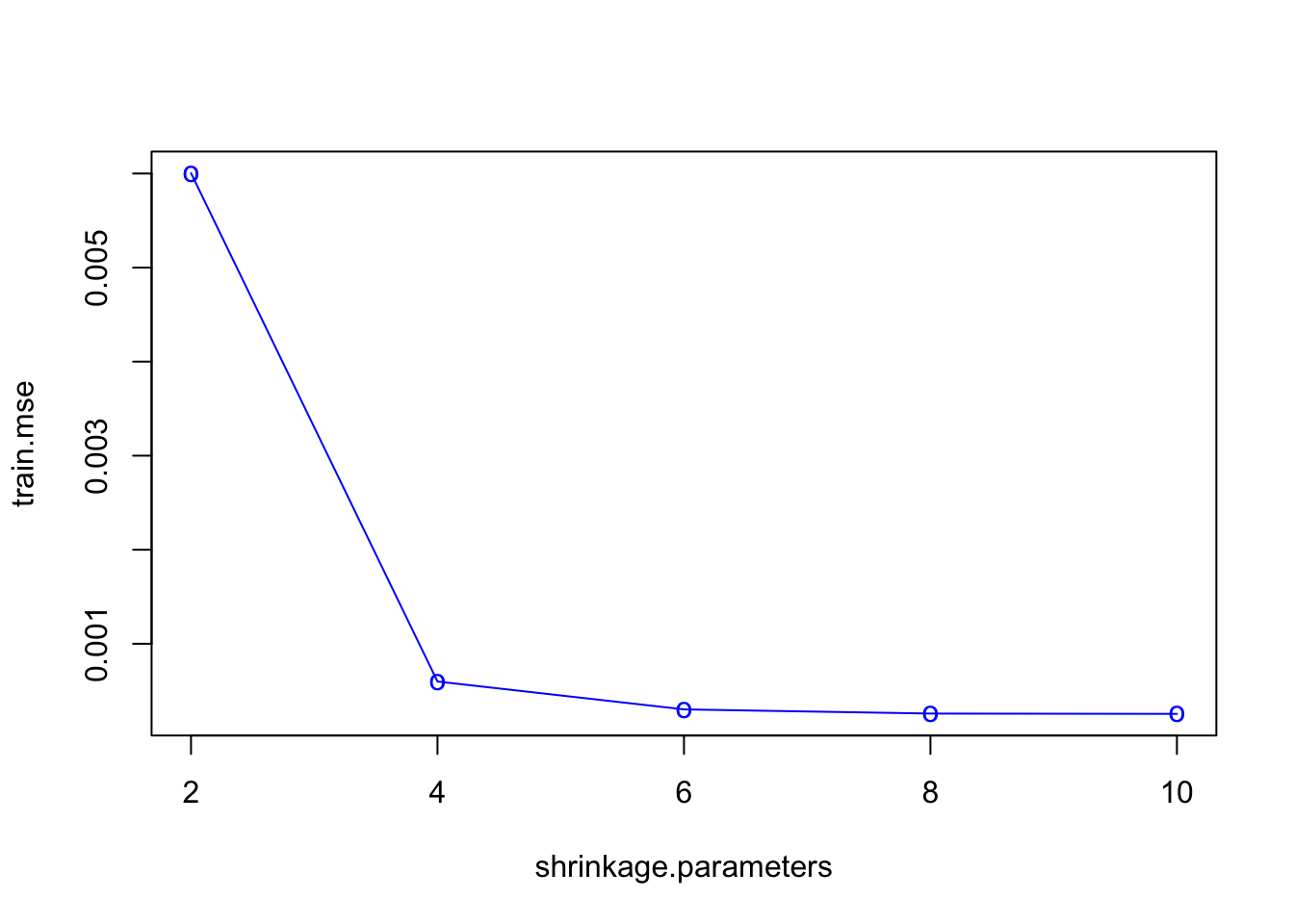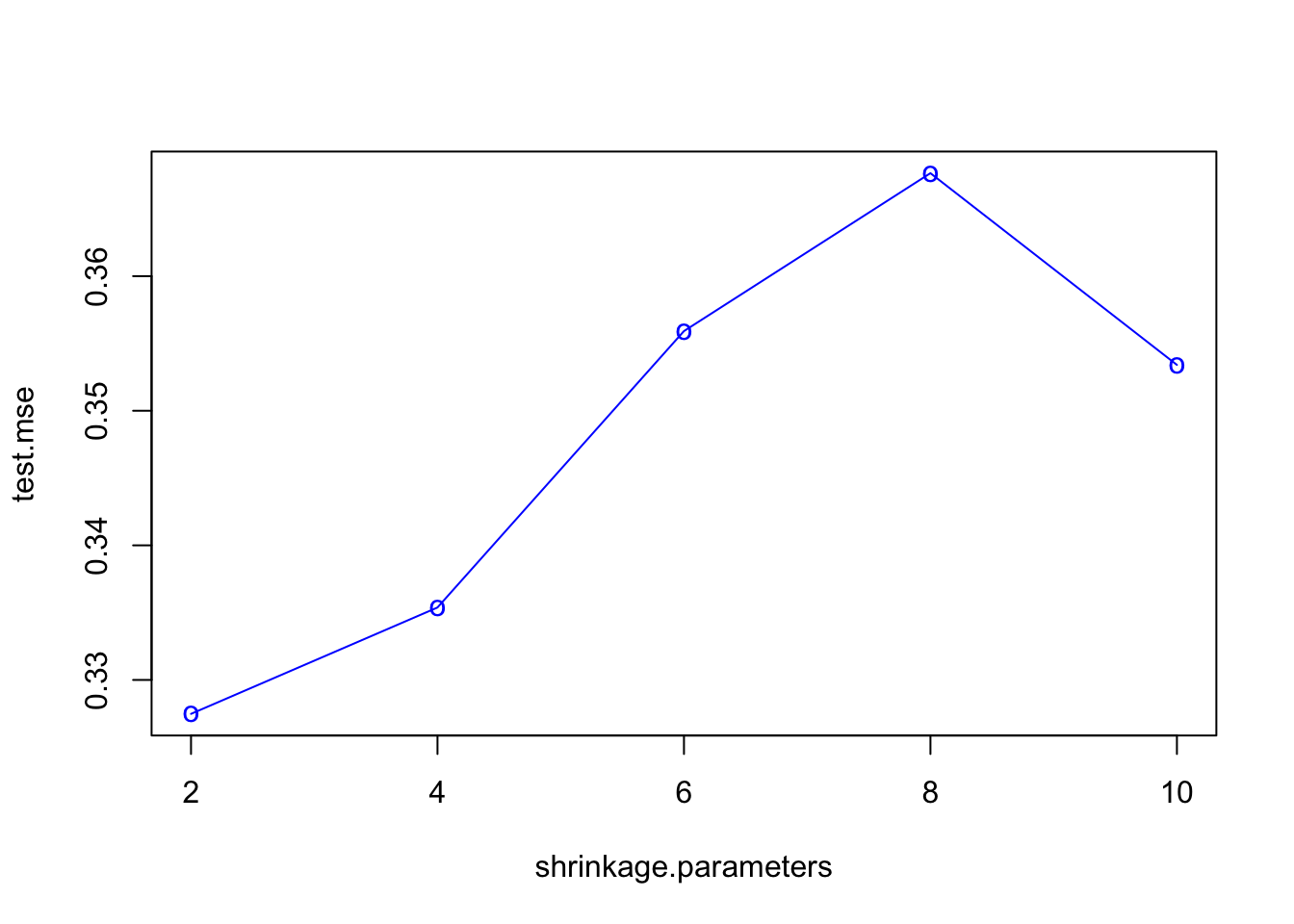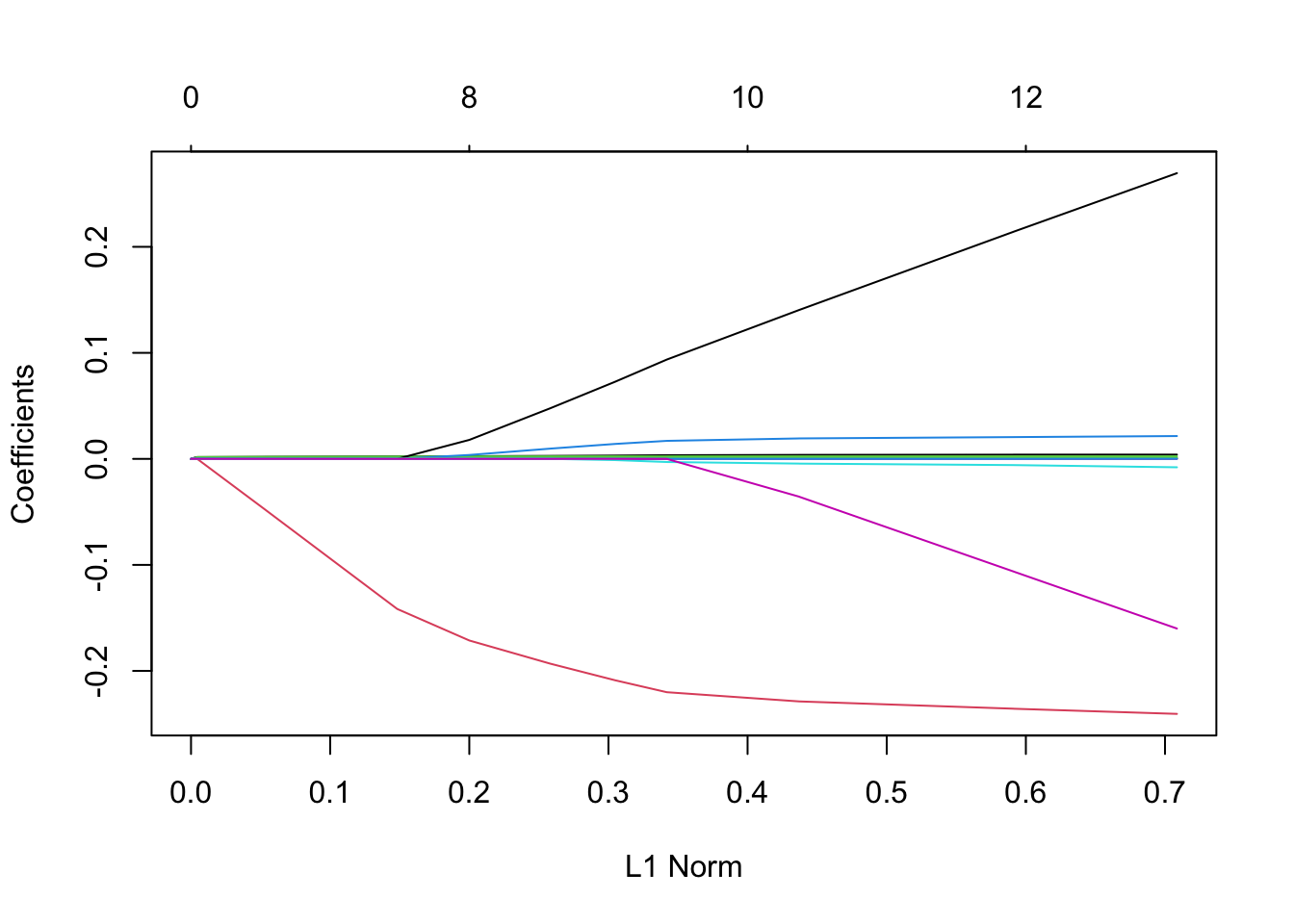ISLR Home

# Question

We now use boosting to predict Salary in the Hitters data set.

1. Remove the observations for whom the salary information is unknown, and then log-transform the salaries.

2. Create a training set consisting of the first 200 observations, and a test set consisting of the remaining observations.

3. Perform boosting on the training set with 1,000 trees for a range of values of the shrinkage parameter λ. Produce a plot with different shrinkage values on the x-axis and the corresponding training set MSE on the y-axis.

4. Produce a plot with different shrinkage values on the x-axis and the corresponding test set MSE on the y-axis.

5. Compare the test MSE of boosting to the test MSE that results from applying two of the regression approaches seen in Chapters 3 and 6.

6. Which variables appear to be the most important predictors in the boosted model?

7. Now apply bagging to the training set. What is the test set MSE for this approach?

``````library(gbm)
library(ISLR)``````

# 10a

## Removing all rows with missing values

``````hitters = na.omit(Hitters)

# Log-transforming the salaries
hitters\$Salary = log1p(hitters\$Salary)``````

# 10b

``````set.seed(1)

# Creating a boolean vector of 200 indicies for train
train = sample(1:nrow(hitters), 200)``````

# 10c

``````# Creating a list of shrinkage parameters
shrinkage.parameters = c(2, 4, 6, 8, 10)

# Training MSE placeholder vector
train.mse = rep(0, length(shrinkage.parameters))

# Test MSE placeholder vector
test.mse = rep(0, length(shrinkage.parameters))

# Fitting a boosting model trying all values in shrinkage.parameters
for (i in 1:length(shrinkage.parameters)) {

# Fitting a boosting model with 1000 trees and shrinkage.parameters[i]
boost.hitters = gbm(
Salary~.,
data=hitters[train,],
distribution="gaussian",
n.trees=1000,
interaction.depth=shrinkage.parameters[i]
)

# Predicting on the train data
yhat.train = predict(boost.hitters, newdata=hitters[train,], n.trees=1000)

# Calculating the train MSE and storing in the train.mse vector
train.mse[i] = mean((yhat.train-hitters[train,]\$Salary)^2)

# Predicting on the test data
yhat.test = predict(boost.hitters, newdata=hitters[-train,], n.trees=1000)

# Calculating test MSE and storing in test,mse vector
test.mse[i] = mean((yhat.test-hitters[-train,]\$Salary)^2)
}``````

## Plotting the training MSE for each shrinkage parameter used

``plot(shrinkage.parameters, train.mse, type="o", col="blue", pch="o", lty=1)``# 10d

COMMENTS: Calculating the test MSE in 10(e)

Plotting the test MSE for each shrinkage parameter used

``plot(shrinkage.parameters, test.mse, type="o", col="blue", pch="o", lty=1)``# 10e

``````# Fitting a Linear Model

## Fitting a linear model on hitters
lm.fit = lm(Salary~., data=hitters, subset=train)

## Predicting on the test set
lm.preds = predict(lm.fit, newdata=hitters[-train,])

## Calculating the MSE of linear model
lm.mse = mean((lm.preds-hitters[-train,]\$Salary)^2)``````

## Fitting a Lasso Model

``library(glmnet)``
``## Loading required package: Matrix``
``## Loaded glmnet 4.0-2``
``````## Preparing the data
x = model.matrix(Salary ~ ., hitters)[,-1]
y = hitters\$Salary

## Creating a grid of lambda values
grid = 10^seq(10, -2, length=100)

## Fitting a Lasso model
lasso.fit = glmnet(
x[train,],
y[train],
alpha=1,
lambda=grid
)``````

## Plotting the results of lasso

``plot(lasso.fit)``
``````## Warning in regularize.values(x, y, ties, missing(ties), na.rm = na.rm):
## collapsing to unique 'x' values``````## Running a CV to determine the best lambda

``````cv.lasso = cv.glmnet(x[train,], y[train], alpha=1)
plot(cv.lasso)``````